CiteScore 2.0
More on impact ›

# Frontiers in Applied Mathematicsand Statistics## ORIGINAL RESEARCH article

Front. Appl. Math. Stat., 16 May 2019 | https://doi.org/10.3389/fams.2019.00024

# Least Square Approach to Out-of-Sample Extensions of Diffusion Maps

• Department of Mathematics and Statistics, Sam Houston State University, Huntsville, TX, United States

Let X = XZ be a data set in ℝD, where X is the training set and Z the testing one. Assume that a kernel method produces a dimensionality reduction (DR) mapping 𝔉: X → ℝd (dD) that maps the high-dimensional data X to its row-dimensional representation Y = 𝔉(X). The out-of-sample extension of dimensionality reduction problem is to find the dimensionality reduction of X using the extension of 𝔉 instead of re-training the whole data set X. In this paper, utilizing the framework of reproducing kernel Hilbert space theory, we introduce a least-square approach to extensions of the popular DR mappings called Diffusion maps (Dmaps). We establish a theoretic analysis for the out-of-sample DR Dmaps. This analysis also provides a uniform treatment of many popular out-of-sample algorithms based on kernel methods. We illustrate the validity of the developed out-of-sample DR algorithms in several examples.

## 1. Introduction

Recently, in many scientific and technological areas, we need to analyze and process high-dimensional data, such as speech signals, images and videos, text documents, stock trade records, and others. Due to the curse of dimensionality [1, 2], directly analyzing and processing high-dimensional data are often infeasible. Therefore, dimensionality reduction (DR) (see the books [3, 4]) becomes a critical step in high-dimensional data processing. DR maps high-dimensional data into a low-dimensional space so that the data process can be carried out on its low-dimensional representation. There exist many DR methods in literature. The famous linear method is principle component analysis (PCA) . However, PCA cannot effectively reduce the dimension for the data set, which essentially resides on a nonlinear manifold. Therefore, to reduce the dimensions of such data sets, people employ non-linear DR methods , among which, the method of Diffusion Maps (Dmaps) introduced by Coifman and his research group [13, 14] have been proved attractive and effective. Adopting the ideas of the spectral clustering [15, 16] and Laplacian eigenmaps , Dmaps integrates them into a more conceptual framework—the geometric harmonics.

As a spectral method, Dmaps employs the diffusion kernel to define the similarity on a given data set X ⊂ ℝD. The principal d-dimensional eigenspace (dD) of the kernel provides the feature space of X, so that a diffusing mapping 𝔉 maps X to the set Y = 𝔉(X), which is called a DR of X.

Note that the mapping 𝔉 is constructed by the spectral decomposition of the kernel, which is data-dependent. If the set X is enlarged to X = XZ and we want to make DR of X by Dmaps, we have to retrain the set X in order to construct a new diffusing mapping. The retraining approach is often unpractical if the cardinality of X becomes very large, or the new data set Z comes as a time-stream.

Out-of-example DR extension method finds the DR of X by extending the diffusing mapping 𝔉 onto X. In most cases, we can assume that the new data set Z has the similar features as X. Therefore, instead of retraining the whole set X, we realize the DR of X by extending the mapping 𝔉 from X to X only.

Lots of papers have introduced various out-of-example extension algorithms (see [14, 18, 19] and their references). However, the mathematical analysis on out-of-example extension is not studied sufficiently.

The main purpose of this paper is to give a mathematical analysis on the out-of-sample DR extension of Dmaps. In Wang , we preliminarily studied out-of-sample DR extensions for kernel PCA. Since the structure of kernels for Dmaps are different from kernel PCA, it needs a special analysis. In this paper we deal with the DR extensions of Dmaps in the framework of reproducing kernel Hilbert space (RKHS), in which Dmaps extension can be classified as the least square one.

The paper is organized as follows: In section 2, we introduce the general out-of-sample extensions in the RKHS framework. In section 3, we establish the least square out-of-sample DR extensions of Dmaps. In section 4, we give the mathematical analysis and algorithms for the Dmaps DR extension. In the last section, we give several examples for the extension.

## 2. Preliminary

We first introduce some notions and notations. Let μ be a finite (positive) measure on a data set X ⊂ ℝD. We denoted by L2(X, μ) the (real) Hilbert space on X, equipped with the inner product

Then, $||f|{|}_{{L}^{2}\left(X,\mu \right)}=\sqrt{{〈f,f〉}_{{L}^{2}\left(X,\mu \right)}}$. Later, we will abbreviate L2(X, μ) to L2(X) (or L2) if the measure μ (and the set X) is (are) not stressed.

Definition 1 A function k: X2 → ℝ is called a Mercer's kernel if it satisfies the following conditions:

1. k is symmetric: k(x, y) = k(y, x);

2. k is positive semi-definite;

3. k is bounded on X2, that is, there is an M > 0 such that |k(x, y)| ≤ M, (x, y) ∈ X2.

In this paper, we only consider Mercer's kernels. Hence, the term kernel will stand for Mercer's one. The kernel distance (associated with k) between two points x, yX is defined by

$dk(x,y)=k(x,x)+k(y,y)-2k(x,y).$

A kernel k defines an RKHS Hk, in which the inner product satisfies 

Later, we will use H instead of Hk if the kernel k is not stressed. Recall that k has a dual identity. It derives the identity operator on H, as shown in 1, and also derives the following compact operator K on L2(X):

In Wang , we proved that if

$k(x,y)=∑j=1mϕj(x)ϕj(y),$

where the set {ϕ1, ··· , ϕm} is linearly independent, then the set is an o.n. basis of H. Therefore, for f, gH with $f=\sum _{j}{c}_{j}{\varphi }_{j}$ and $g=\sum _{j}{d}_{j}{\varphi }_{j}$, we have ${〈f,g〉}_{{H}_{k}}=\sum _{j}{c}_{j}{d}_{j}$.

Let the spectral decomposition of k be the following:

where the eigenvalues are arranged decreasingly, λ1 ≥ ··· ≥ λm > 0, and the eigenfunctions v1, v1, ··· , vm, are normalized to satisfy

$〈vi,vj〉L2(X)=δi,j.$

Write ${\gamma }_{i}\left(x\right)=\sqrt{{\lambda }_{i}}{v}_{i}\left(x\right)$. Then, {γ1, ··· , γm} is an o.n. basis of H, which is called the canonic basis of H. We also call $k\left(x,y\right)=\sum _{j=1}^{m}{\gamma }_{j}\left(x\right){\gamma }_{j}\left(y\right)$ the canonic decomposition of k. By 2, we have

$γj=1λj∫Xk(x,y)γj(y)dμ(y).$

Thus, if fH have the canonic representation $f=\sum _{j=1}^{m}{c}_{j}{\gamma }_{j}$, then, for any gH, the inner product 〈f, gH has the following integral form:

$〈f,g〉H=∑j=1mcjλj∫Xg(x)γj(x)dμ(x).$

To investigate the out-of-sample DR extension, we first recall some general results on function extensions. Let X = XZ. To stress that a point xX is also in X, we use x instead of x. Similarly, we denote by k(x, y) the restriction of k(x, y) on X2. That is,

We also denote by H the RKHS associated with k. Then a continuous map E : HH is called an extension if

Correspondingly, we define the restriction R: HH by

It is obvious that the extensions from X to X are not unique if Z is not empty. So, we define the set of all extensions of fH by

and call $\stackrel{^}{f}\in {A}_{\text{f}}$ the least-square extension of f if

$||f^||H=minf∈Af||f||H.$

It is evident that the least-square extension of a function is unique. We denote by T : HH the operator of the least-square extension.

In Wang , we already prove the following:

1. Let {v1, ··· , vd} be the canonic basis of H and σ1 ≥ σ2 ≥ ··· ≥ σd > 0 be the eigenvalues of the kernel k(x, y). Then the least-square extension of vj is

Therefore, for any $\text{f}=\sum _{j=1}^{d}{c}_{j}{\text{v}}_{j}\in \text{H}$,

$f^(x)(=T(f)(x))=∑j=1dcj1σj∫Xk(x,y)vj(y)dμ(y).$

2. Let Ĥ = T(H) and T* : HH be the joint operator of T. Then P = TT* is an orthogonal projection from H to Ĥ.

3. Let $\stackrel{^}{k}\left(x,y\right)$ be the kernel of the RKHS Ĥ. Then ${k}_{0}\left(x,y\right)=k\left(x,y\right)-\stackrel{^}{k}\left(x,y\right)$ is a Mercer's kernel such that ${k}_{0}\left(x,y\right)=0,\left(x,y\right)\in {X}^{2}\{\text{X}}^{2}$. Denote by H0 the RKHS associated with k0. Then, H = Ĥ ⊕ H0 and Ĥ ⊥ H0.

4. If k(x, y) is a Gramian-type DR kernel , and ${\left[{\text{v}}_{1}\left(\text{X}\right),···\phantom{\rule{0.3em}{0ex}},{\text{v}}_{d}\left(\text{X}\right)\right]}^{T}$ gives the DR of X, then ${\left[{\stackrel{^}{v}}_{1}\left(X\right),···\phantom{\rule{0.3em}{0ex}},{\stackrel{^}{v}}_{d}\left(X\right)\right]}^{T}$ provides the least-square out-of-sample DR extension on X.

## 3. Least-Square Out-of-Sample DR Extensions for Dmaps

The kernels of Dmaps are constructed based on the Gaussian kernel

The function

$S(x)=∫Xw(x,y)dμ(y)$

defines a mass density on X, and $M={\int }_{X}S\left(x\right)d\mu \left(x\right)$ is the total mass of X.

There are two important forms of the kernels of Dmaps: The Graph-Laplacian diffusion kernel and the Laplace-Beltrami one.

### 3.1. Dmaps With the Graph-Laplacian Kernel

We first discuss the least-square out-of-sample DR Extensions for the Dmaps with the Graph-Laplacian (GL) kernel. Normalizing the Gaussian kernel by S(x), we obtain the following Graph-Laplacian diffusion kernel [4, 13]:

$g(x,y)=w(x,y)S(x)S(y).$

This kernel relates to the data set X equipped with an undirected (weighted) graph. It is known that 1 is the greatest eigenvalue of g(x, y) and its corresponding normalized eigenfunction is $\sqrt{\frac{S\left(x\right)}{M}}$.

Let Hg be the RKHS associated with the kernel g and {ϕ0, ··· , ϕm} be its canonic basis, which suggest the following spectral decomposition of g(x, y):

$g(x,y)=∑j=0mλjvj(x)vj(y),$

where 1 = λ0 ≥ λ1 ≥ ··· ≥ λm > 0 and ${v}_{j}\left(x\right)={\varphi }_{j}\left(x\right)/\sqrt{{\lambda }_{j}}$. Because ${\varphi }_{0}=\sqrt{\frac{S\left(x\right)}{M}}$ provides only the mass information of the data set, it should not reside on the feature space. Hence, we define the feature space as the RKHS associated with the kernel $\sum _{j=1}^{m}{\varphi }_{j}\left(x\right){\varphi }_{j}\left(y\right)$, where ϕ0 is removed.

Definition 2. The mapping $\Phi :X\to {ℝ}^{m}:\Phi \left(x\right)={\left[{\varphi }_{1}\left(x\right),···\phantom{\rule{0.3em}{0ex}},{\varphi }_{m}\left(x\right)\right]}^{T}$ is called the diffusion mapping and the data set Φ(X) ⊂ ℝm is called a DR of X.

Remark. In Wang , we already pointed out that each orthogonal transformation of the set Φ(X) can also be considered as a DR of X. Hence, any non-canonical o.n. basis of the feature space also provides a DR mapping.

To study the out-of-sample extension, as what was done in the preceding section, we assume X = XZ and denote by g(x, y) the Graph-Laplacian kernel on X, that is,

$g(x,y)=w(x,y)S(x)S(y),$

where S(x) is the mass density on X, and

Assume that spectral decomposition of g is given by

Then the RKHS Hg associated with g has the canonic basis {φ0, φ1, ··· , φd}:

$g(x,y)=∑j=0dφj(x)φj(y),$

where ${\phi }_{j}=\sqrt{{\sigma }_{j}}{\text{v}}_{j}$. Because S(x) ≠ S(x), in general,

Hence, we cannot directly apply the extension technique in the preceding section to g. Our main purpose in this subsection is to introduce the extension from Hg to Hg.

Denote by Hw and Hw the RKHSs associated with the kernels w and w, respectively. Because w(x, y) = w(x, y) for (x, y) ∈ X2, the extension technique in the preceding section can be applied.

Let ${\text{u}}_{j}\left(\text{x}\right)=\sqrt{\text{S}\left(\text{x}\right)}{\phi }_{j}\left(\text{x}\right)$ and ${u}_{j}\left(x\right)=\sqrt{S\left(x\right)}{\varphi }_{j}\left(x\right)$. Then we have

Lemma 3 The least-square extension operator T : HwHw has the following representation:

Proof. Because ${\left\{{\text{u}}_{j}\right\}}_{j=0}^{d}$ is not a canonic o.n. basis of Hw, we cannot directly apply the extension formula 3. Recall that the formula 3 can also be written as T(f)(x) = 〈f, k(x, ·)〉H. (In the considered case, the kernel w replaces k.) Note that

which implies that, for any fHw, we have

$〈f,uj〉Hw=1σj∫Xf(y)uj(y)S(y)dμ(y).$

Therefore, the formula T(uj)(x) = 〈w(x, ·),ujHw yields 5. ■

We now write ûj = T(uj) and define

$ŵ(x,y)=∑j=0dûj(x)ûj(y).$

Then the RKHS Hŵ associated with the kernel ŵ is the extension of Hw.

The function S(x) induces the following multiplicator from Hg to Hw:

Similarly, the function S(x) induces the following multiplicator from Hg to Hw:

It is clear that the operator 𝔖S (𝔖S) is an isometric mapping. With the aid of 𝔖S and 𝔖S, we define the least-square extension ${T}$ from Hg to Hg by

The following diagram shows the strategy of the out-of-sample extension using Graph-Laplacian diffusion mapping.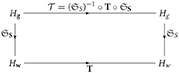We now derive the integral representation of the operator ${T}$.

Lemma 4 Let the canonic decomposition of g be given by 4 and $\text{f}=\sum _{j=0}^{d}{c}_{j}{\phi }_{j}\in {H}_{\text{g}}$. Then

Its adjoint operator ${{T}}^{*}:{H}_{g}\to {H}_{\text{g}}$ is given by

Proof. Write ${\stackrel{^}{\varphi }}_{j}={T}\left({\phi }_{j}\right)$. By 6, we have ${\stackrel{^}{\varphi }}_{j}\left(x\right)=\frac{\text{T}\left({\text{u}}_{j}\right)\left(x\right)}{\sqrt{S\left(x\right)}}.$ By Lemma 3, we obtain

which yields 7. Recall that $\frac{w\left(x,\text{y}\right)}{\sqrt{S\left(x\right)\text{S}\left(\text{y}\right)}}=g\left(x,\text{y}\right)\sqrt{\frac{S\left(\text{y}\right)}{\text{S}\left(\text{y}\right)}}$. For any hHg, by 〈h, g(·, y)〉Hg = h(y), we have

$〈h,T(f)〉Hg=∑j=0dcjσj∫Xh(y)S(y)S(y)φj(y)dμ(y)=〈S(·)S(·)h(·),f〉Hg,$

which yields 8. ■

We now give the main theorem in this subsection.

Theorem 5 Let ${T}$ be the operator defined in 6. Define $ĝ\left(x,y\right)=\sum _{j=0}^{d}{\stackrel{^}{\varphi }}_{j}\left(x\right){\stackrel{^}{\varphi }}_{j}\left(y\right)$, where ${\stackrel{^}{\varphi }}_{j}={T}\left({\phi }_{j}\right)$, and let Hĝ be the RKHS associated with ĝ. Then,

(i) ${{T}}^{*}{T}=I$ on Hg.

(ii) $\left\{{\stackrel{^}{\varphi }}_{0},···\phantom{\rule{0.3em}{0ex}},{\stackrel{^}{\varphi }}_{d}\right\}$ is an orthonormal system in Hg, so that Hĝ is a subspace of Hg and ${P}={T}{{T}}^{*}$ is an orthogonal projection from Hg to Hĝ. Therefore, we have ${P}\left({\stackrel{^}{\varphi }}_{j}\right)={\stackrel{^}{\varphi }}_{j}$ and ${{T}}^{*}\left({\stackrel{^}{\varphi }}_{j}\right)={\phi }_{j}$.

(iii) The function g0(x, y) = g(x, y) − ĝ(x, y) is a Mercer's kernel. The RKHS Hg0 associated with g0 is (md) dimensional. Besides, Hg = HĝHg0 and HĝHg0.

(iv) For any function fHg0, f(x) = 0, xX.

Proof. Recall that {φ0, φ1, ··· , φd} is an on. basis of Hg. By 8 and 9, we have

which yields ${{T}}^{*}{T}\left({\phi }_{j}\right)={\phi }_{j}$. Hence, ${{T}}^{*}{T}=I$ on Hg. The proof of (i) is completed.

Note that

$〈ϕ^i,ϕ^j〉Hg=〈φi,T*T(φj)〉Hg=〈φi,φj〉Hg=δi,j,$

which indicates that $\left\{{\stackrel{^}{\varphi }}_{0}\left(x\right),···\phantom{\rule{0.3em}{0ex}},{\stackrel{^}{\varphi }}_{d}\left(x\right)\right\}$ is an orthonormal system in Hg and Hĝ is a subspace of Hg. Because ${{P}}^{2}={P}$ and ${P}\left({\stackrel{^}{\varphi }}_{j}\right)={\stackrel{^}{\varphi }}_{j},j=0,1,···\phantom{\rule{0.3em}{0ex}},d$, ${P}$ is an orthogonal projection from ${H}_{\stackrel{~}{g}}$ to Hĝ, which proves (ii).

It is clear that (iii) is a direct consequence of (ii). Finally, we have ${P}\left(f\right)=0$ for fHg0, which yields ${{T}}^{*}\left(f\right)=0$. Therefore, f(x) = 0, xX. The proof of (iv) is completed, ■

By Definition 2, the mapping $\Phi :\Phi \left(\text{x}\right)={\left[{\phi }_{1}\left(\text{x}\right),···\phantom{\rule{0.3em}{0ex}},{\phi }_{d}\left(\text{x}\right)\right]}^{T}$ is a diffusion mapping from X to ℝd and the set Φ(X) is a DR of X. We now give the following definition.

Definition 6 Let ${T}$ be the operator defined in 6 and ${\stackrel{^}{\varphi }}_{j}={T}\left({\phi }_{j}\right)$. Then the set $\stackrel{^}{\Phi }\left(X\right)={\left[{\stackrel{^}{\varphi }}_{1}\left(X\right),···\phantom{\rule{0.3em}{0ex}},{\stackrel{^}{\varphi }}_{d}\left(X\right)\right]}^{T}\subset {ℝ}^{d}$ is called the least-square out-of-sample DR extension of the Dmaps with the Graph-Laplacian kernel.

A DR extension on X is called exact if it is equal to a DR of X as defined in Definition 2 (see ). The following corollary is a direct consequence of Theorem 5.

Corollary 7 The least-square out-of-sample DR extension given by ${T}$ from Hg to Hg is exact if and only if dim(Hg) = dim(Hg), or equivalently, Hg0 = {0}.

### 3.2. Dmaps With the Laplace-Beltrami Kernel

The discussion on the out-of-sample DR extension of Dmaps with the Laplace-Beltrami (BL) kernel is similar to that in the previous subsection. Hence, in this subsection, we only outline the main results, skipping the details. We start the discussion from the asymmetrically normalized kernel

$m(x,y)=1S(x)w(x,y),$

which defines a random walk on the data set X such that m(x, y) is the probability of the walk from the node x to the node y after a unit time. From the viewpoint of the random walk, we naturally modify the Gaussian kernel w(x, y) to the following:

$a(x,y)=w(x,y)S(x)S(y).$

Then, we normalize it to

$b(x,y)=a(x,y)P(x)P(y)=w(x,y)R(x)R(y),$

where

We call b(x, y) the Laplace-Beltrami kernel of Dmaps, which relates to the data set X sampled from a manifold in ℝD. The greatest eigenvalue of b(x, y) is also 1, which corresponds to the normalized eigenfunction $\sqrt{\frac{P\left(x\right)}{L}}$, where

$L=∫XP(x)dμ(x).$

Let Hb be the RKHS associated with b and assume that the spectral decomposition of b is

$b(x,y)=∑j=0mβjqj(x)qj(y)=∑j=0mψj(x)ψj(y),$

where 1 = β0 ≥ β1 ≥ ··· ≥ βm > 0 and ${\psi }_{j}\left(x\right)=\sqrt{{\beta }_{j}}{q}_{j}\left(x\right)$. Similar to the discussion in the previous subsection, since ${\psi }_{0}=\sqrt{\frac{P\left(x\right)}{L}}$ does not contains any feature of the data set, we exclude it from the feature space.

Definition 8 The mapping $\text{Ψ}:X\to {ℝ}^{m}:\text{Ψ}\left(x\right)={\left[{\psi }_{1}\left(x\right),···\phantom{\rule{0.3em}{0ex}},{\psi }_{m}\left(x\right)\right]}^{T}$ is called the Laplace-Beltrami diffusion mapping and the data set Ψ(X) ⊂ ℝm is called a DR of X associated with Laplace-Beltrami Dmaps.

We new assume again X = XZ and denote by b(x, y) the Laplace-Beltrami kernel on X. Assume that spectral decomposition of b is

Then the RKHS Hb associated with b has the canonic basis {ω0, ω1, ··· , ωd}, where ${\omega }_{j}=\sqrt{{\gamma }_{j}}{\text{q}}_{j}$.

Define the multiplicator from Hb to Hw by

and the multiplicator from Hb to Hw by

The operator 𝔖R (𝔖R) is an isometric mapping. We now define the least-square extension ${M}$ from Hb to Hb by

The integral representation of ${M}$ is give by the following lemma:

Lemma 9 Let0, ω1, ··· , ωd}be the canonic basis of b. Write ${\stackrel{^}{\psi }}_{j}={M}\left({\omega }_{j}\right)$. Then

Particularly, for $\text{f}=\sum _{j=0}^{d}{c}_{j}{\omega }_{j}\in {H}_{\text{b}}$, we have

$M(f)(x)=∑j=0dcjψ^j(x)=∑j=0dcjγj∫Xw(x,y)R(x)R(y)ωj(y)dμ(y).$

Its adjoint operator ${{M}}^{*}:{H}_{b}\to {H}_{\text{b}}$ is given by

Since the proof is similar to that for Lemma 4, we skip it here.

Theorem 10 Let ${M}$ be the operator defined in 11. Define $\stackrel{^}{b}\left(x,y\right)=\sum _{j=0}^{d}{\stackrel{^}{\psi }}_{j}\left(x\right){\stackrel{^}{\psi }}_{j}\left(y\right)$, where ${\stackrel{^}{\psi }}_{j}={M}\left({\omega }_{j}\right)$, and let ${H}_{\stackrel{^}{b}}$ be the RKHS associated with $\stackrel{^}{b}$. Then,

1. ${{M}}^{*}{M}=I$ on Hb.

2. $\left\{{\stackrel{^}{\psi }}_{0},···\phantom{\rule{0.3em}{0ex}},{\stackrel{^}{\psi }}_{d}\right\}$ is an orthonormal system in Hb, so that ${H}_{\stackrel{^}{b}}$ is a subspace of Hb and ${Q}={M}{{M}}^{*}$ is an orthogonal projection from Hb to ${H}_{\stackrel{^}{b}}$. Therefore, we have ${Q}\left({\stackrel{^}{\psi }}_{j}\right)={\stackrel{^}{\psi }}_{j}$ and ${{M}}^{*}\left({\stackrel{^}{\psi }}_{j}\right)={\omega }_{j}$.

3. The function ${b}_{0}\left(x,y\right)=b\left(x,y\right)-\stackrel{^}{b}\left(x,y\right)$ is a Mercer's kernel. The RKHS Hb0 associated with b0 is (md) dimensional. Besides, ${H}_{b}={H}_{\stackrel{^}{b}}\oplus {H}_{{b}_{0}}$ and ${H}_{\stackrel{^}{b}}\perp {H}_{{b}_{0}}$.

4. For any function fHb0, f(x) = 0, xX.

We skip the proof of Theorem 10 because it is similar to that for Theorem 5. We now give the following definition:

Definition 11 Let ${M}$ be the operator defined in 11 and ${\stackrel{^}{\psi }}_{j}={M}\left({\omega }_{j}\right)$. Then the set $\stackrel{^}{\text{Ψ}}\left(X\right)={\left[{\stackrel{^}{\psi }}_{1}\left(X\right),···\phantom{\rule{0.3em}{0ex}},{\stackrel{^}{\psi }}_{d}\left(X\right)\right]}^{T}\subset {ℝ}^{d}$ is called the least-square out-of-sample DR extension of the Dmaps with the Laplace-Beltrami kernel.

Corollary 12 The least-square out-of-sample DR extension given by ${M}$ from Hb to Hb is exact if and only if dim(Hb) = dim(Hb), or equivalently, Hb0 = {0}.

### 3.3. Algorithms for Out-of-Sample DR Extension of Dmaps

In this subsection, we present the algorithm for out-of-sample DR extension of Dmaps. The algorithm contains two parts. In the first part, we construct the DR for X by 4 and 10. In the second part, we extend the DR to the set X, by 9 and 12.

In the algorithm, we represent the data sets X, Z, and X = XZ as the D×N, D×M, and D×(N+M) matrices, respectively, so that X = [X, Z]. We assume the measure (x) = dx. Write X = [x1, ··· , xN], Z = [z1, ··· , zM], and X = [x1, ··· , x(N+M)], where xj = xj, 1 ≤ jN and xj = zjN, N + 1 ≤ jN + M. Then we represent all kernels by matrices and all functions by vectors. For example, w is now represented by the N × N matrix with ${\text{w}}_{i,j}=exp\left(-||{\text{x}}_{i}-{\text{x}}_{j}|{|}^{2}/ϵ\right)$. To treat GL-map and LB-map in a uniform way, we write ${\text{S}}_{i}=\sum _{j}{\text{w}}_{i,j}$ and define

Then we set either kernel on X as the N × N matrix k with

$ki,j=wi,jdidj.$

The pseudo-code is given in Algorithm 1.

## 4. Illustrative Examples

In this section, we give several illustrative examples to show the validity of the Dmaps out-of-sample extensions. We employ four benchmark artificial data sets, S-curve, Swiss roll, punched sphere, and 3D cluster, in our samples. The graphs of these four data sets are give in Figure 1.

FIGURE 1

### 4.1. Out-of-Sample Extension by Graph-Laplacian Mapping

We first show the examples for the out-of-sample extensions provided by Graph-Laplacian mapping for the four benchmark figures. We set the size of each of these data sets by |X| = 2, 048. When the out-of-example algorithm is applied, we choose the size of the training data set to be |X| = 1, 843, which is 90% of the all samples, and choose the size of the testing set |Z| = 205, which is 10% of all samples. The parameters for the Graph-Laplacian kernel are set as follows: For obtaining the sparse kernel, we choose 25 nearest neighbors for every node, and assign the diffusion parameter ϵ = 1 for S-curve, Punched Sphere, and 3D Cluster, while assign ϵ = ∞ for Swiss Roll. We compare the DR result of the whole set X obtained by out-of-example extension with that obtained without out-of-example extension in the Figures 25. The figures show that the DRs obtained by out-of-sample extensions are satisfactory.

FIGURE 2
FIGURE 3
FIGURE 4
FIGURE 5

### 4.2. Out-of-Sample Extension by Laplace-Beltrami Mapping

We now show the examples for the out-of-sample extensions provided by Laplace-Beltrami mapping for the same four benchmark figures. We set the same sizes for |X|,|X|, and |Z|, respectively. The parameters for the Laplace-Beltrami kernel are also set the same as for Graph-Laplacian kernel. The results of the comparisons are give in Figures 69.

FIGURE 6
FIGURE 7
FIGURE 8
FIGURE 9

To give more detailed comparisons, in Figures 1013, we show the DRs of the training data and the testing data obtained by out-of-extensions and without extensions, respectively, for LB mapping.

FIGURE 10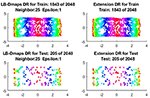Figure 10. Comparisons of DRs of training data and the testing data, respectively, for S-curve.

FIGURE 11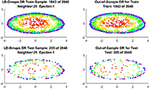Figure 11. Comparisons of DRs of training data and the testing data, respectively, for Punched Sphere.

FIGURE 12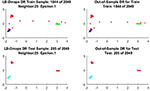Figure 12. Comparisons of DRs of training data and the testing data, respectively, for 3D Cluster.

FIGURE 13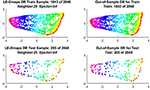Figure 13. Comparisons of DRs of training data and the testing data, respectively, for Swiss Roll.

It is a common sense that if we reduce the size of the training set while increase the size for the testing set, the out-of-sample extension will introduce larger errors for DR. Figures 1415 show that, in a relative large scope, say, the size of the testing set is no greater than the size of the training set. the out-of-sample extension still produces the acceptable results.

FIGURE 14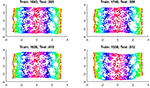Figure 14. LB out-of-sample extension for different sizes of the test sets of S-curve (I).

FIGURE 15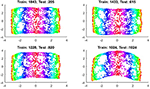Figure 15. LB out-of-sample extension for different sizes of the test sets of S-curve (II).

## Data Availability

No datasets were generated or analyzed for this study.

## Author Contributions

The author confirms being the sole contributor of this work and has approved it for publication.

## Conflict of Interest Statement

The author declares that the research was conducted in the absence of any commercial or financial relationships that could be construed as a potential conflict of interest.

## References

1. Bellman R. Adaptive Control Processes: A Guided Tour. Princeton, NJ: Princeton University Press (1961).

2. Scott DW, Thompson JR. Probability density estimation in higher dimensions. In: Gentle JE, editor. Computer Science and Statistics: Proceedings of the Fifteenth Symposium on the Interface. Amsterdam; New York, Ny; Oxford: North Holland-Elsevier Science Publishers (1983). p. 173–9.

3. Lee JA, Verleysen M. Nonlinear Dimensionality Reduction. New York, NY: Springer (2007).

4. Wang JZ. Geometric Structure of High-Dimensional Data and Dimensionality Reduction. Beijing; Berlin; Heidelberg: Higher Educaiton Press; Springer (2012).

5. Jolliffe IT. Principal Component Analysis. Springer Series in Statistics. Berlin: Springer-Verlag (1986).

6. Zhang ZY, Zha HY. Principal manifolds and nonlinear dimensionality reduction via local tangent space alignment. SIAM J Sci Comput. (2004) 26:313–38.

7. Schölkopf B, Smola A, Müller K-R. Nonlinear component analysis as a kernel eigenvalue problem. Neural Comput. (1998) 10:1299–319.

8. Roweis ST, Saul LK. Nonlinear dimensionality reduction by locally linear embedding. Science. (2000) 290:2323–6. doi: 10.1126/science.290.5500.2323

9. Donoho DL, Grimes C. Hessian eigenmaps: new locally linear embedding techniques for high-dimensional data. Proc Natl Acad Sci USA. (2003) 100:5591–6. doi: 10.1073/pnas.1031596100

10. Shmueli Y, Sipola T, Shabat G, Averbuch A. Using affinity perturbations to detect web traffic anomalies. In: The 11th International Conference on Sampling Theory and Applications (Bremen) (2013).

11. Shmueli Y, Wolf G, Averbuch A. Updating kernel methods in spectral decomposition by affinity perturbations. Linear Algebra Appl. (2012) 437:1356–65. doi: 10.1016/j.laa.2012.04.035

12. Balasubramanian M, Schwaartz E, Tenenbaum J, de Silva V, Langford J. The isomap algorithm and topological staility. Science (2002) 295:7. doi: 10.1126/science.295.5552.7a

13. Coifman RR, Lafon S. Diffusion maps. Appl Comput Harmon Anal. (2006) 21:5–30. doi: 10.1016/j.acha.2006.04.006

14. Coifman RR, Lafon S. Geometric harmonics: a novel tool for multiscale out-of-sample extension of empirical functions. Appl Comput Harmon Anal. (2006) 2:31–52. doi: 10.1016/j.acha.2005.07.005

15. Ng A, Jordan M, Weiss Y. On spectral clustering: analysis and an algorithm. Adv Neural Inform Process Syst. (2001) 14:849–56.

16. Shi J, Malik J. Normalized cuts and image segmentation. IEEE Trans Pattern Anal Mach Intell. (2000) 22:888–905. doi: 10.1109/34.868688

17. Belkin M, Niyogi P. Laplacian eigenmaps for dimensionality reduction and data representation. Neural Comput. (2003) 15:1373–96. doi: 10.1162/089976603321780317

18. Aizenbud Y, Bermanis A, Averbuch A. PCA-based out-of-sample extension for dimensionality reduction. arXiv: 1511.00831 (2015).

19. Bengio Y, Paiement J, Vincent P, Delalleau O, Le Roux N, Ouimet M. Out-of-sample extensions for LLE, Isomap, MDS, eigenmaps, and spectral clustering. In: Thrun S, Saul L, Schölkopf B, editors. Advances in Neural Information Processing Systems. Cambridge, MA: MIT Press (2004).

20. Wang JZ. Mathematical analysis on out-of-sample extensions. Int J Wavelets Multiresol Inform Process. (2018) 16:1850042. doi: 10.1142/S021969131850042X

21. Aronszajn N. Theory of reproducing kernels. Trans Amer Math Soc. (1950) 68:337–404.

Keywords: out-of-sample extension, dimensionality reduction, reproducing kernel Hilbert space, least-square method, diffusion maps

AMS Subject Classification: 62-07, 42B35, 47A58, 30C40, 35P15

Citation: Wang J (2019) Least Square Approach to Out-of-Sample Extensions of Diffusion Maps. Front. Appl. Math. Stat. 5:24. doi: 10.3389/fams.2019.00024

Received: 02 March 2019; Accepted: 25 April 2019;
Published: 16 May 2019.

Edited by:

Ding-Xuan Zhou, City University of Hong Kong, Hong Kong

Reviewed by:

Bo Zhang, Hong Kong Baptist University, Hong Kong
Shao-Bo Lin, Wenzhou University, China
Sui Tang, Johns Hopkins University, United States

Copyright © 2019 Wang. This is an open-access article distributed under the terms of the Creative Commons Attribution License (CC BY). The use, distribution or reproduction in other forums is permitted, provided the original author(s) and the copyright owner(s) are credited and that the original publication in this journal is cited, in accordance with accepted academic practice. No use, distribution or reproduction is permitted which does not comply with these terms.

*Correspondence: Jianzhong Wang, jzwang@shsu.edu